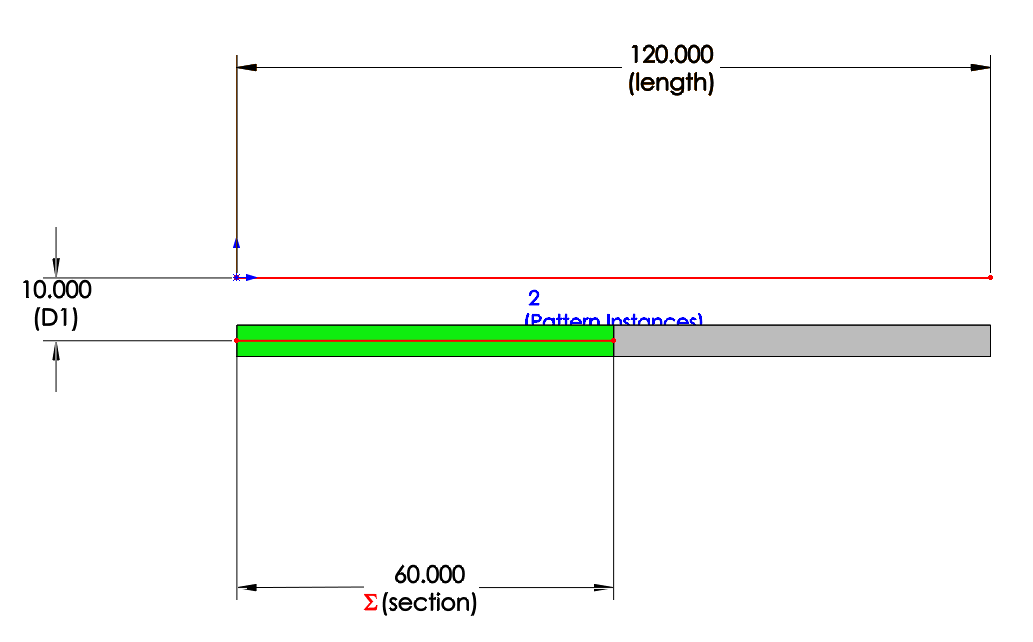# Nested IF Statements in SOLIDWORKS Equations to control dimension lengthArticle by Jamie Hill, CSWE updated May 24, 2018

###### Article

Recently a customer asked me:

“Is it possible to automatically size sections of a body or component based on an overall length?”

This stood out to me as a perfect scenario to utilize the power of equations, and more specifically nested SOLIDWORKS IF statements.

### IF Statement example

The max section length permitted is 119″ and all sections must be equal in length based on the overall length. Therefore if the overall length was 119″, there would only be one section, if the overall length was 120″, there would be 2 sections at 60″ and so on…Example for IF statement

Here is the equation I used

“section@Sketch1” = ROUND ( IIF ( “length@Sketch1” < = 119 , “length@Sketch1” / 1 , IIF ( “length@Sketch1” < = 238 , “length@Sketch1” / 2 , IIF ( “length@Sketch1” < = 357 , “length@Sketch1” / 3 , 120 ) ) ) , 2 )

You’ll notice I’ve also incorporated a 2 decimal rounding function to take care of those pesky repeating decimal values.

#### Get Certified SOLIDWORKS Services from Javelin